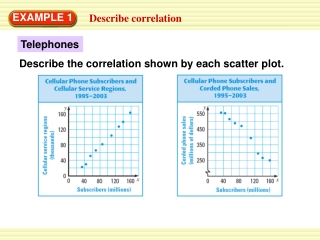DownloadDownload PresentationDescribe correlation

# Describe correlation

Télécharger la présentation## Describe correlation

- - - - - - - - - - - - - - - - - - - - - - - - - - - E N D - - - - - - - - - - - - - - - - - - - - - - - - - - -
##### Presentation Transcript

1. EXAMPLE 1 Describe correlation Telephones Describe the correlation shown by each scatter plot.

2. EXAMPLE 1 Describe correlation SOLUTION The first scatter plot shows a positive correlation, because as the number of cellular phone subscribers increased, the number of cellular service regions tended to increase. The second scatter plot shows a negative correlation, because as the number of cellular phone subscribers increased, corded phone sales tended to decrease.

3. a. a. The scatter plot shows a clear but fairly weak negative correlation. So, r is between 0 and –1, but not too close to either one. The best estimate given is r=–0.5. (The actual value is r–0.46.) EXAMPLE 2 Estimate correlation coefficients Tell whether the correlation coefficient for the data is closest to –1, –0.5, 0, 0.5, or 1. SOLUTION

4. b. b. The scatter plot shows approximately no correlation. So, the best estimate given is r=0. (The actual value is r –0.02.) EXAMPLE 2 Estimate correlation coefficients SOLUTION

5. c. c. The scatter plot shows a strong positive correlation. So, the best estimate given is r = 1. (The actual value is r0.98.) EXAMPLE 2 Estimate correlation coefficients SOLUTION

6. 1. for Examples 1 and 2 GUIDED PRACTICE For each scatter plot, (a) tell whether the data have a positive correlation, a negative correlation, or approximately no correlation, and (b) tell whether the correlation coefficient is closest to –1, – 0.5, 0, 0.5, or 1. ANSWER (a)positive correlation (b)r = 0.5

7. 2. for Examples 1 and 2 GUIDED PRACTICE For each scatter plot, (a) tell whether the data have a positive correlation, a negative correlation, or approximately no correlation, and (b) tell whether the correlation coefficient is closest to –1, – 0.5, 0, 0.5, or 1. ANSWER (a)negative correlation (b)r = –1

8. 3. for Examples 1 and 2 GUIDED PRACTICE For each scatter plot, (a) tell whether the data have a positive correlation, a negative correlation, or approximately no correlation, and (b) tell whether the correlation coefficient is closest to –1, –0.5, 0, 0.5, or 1. ANSWER (a)no correlation (b)r = 0